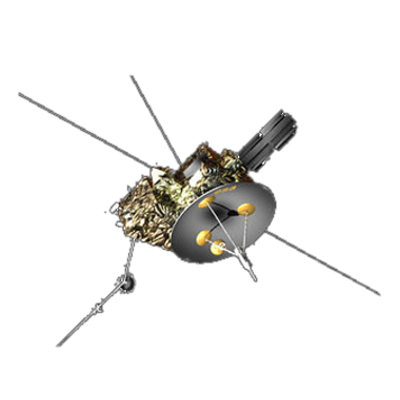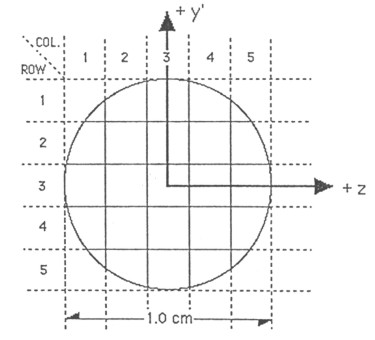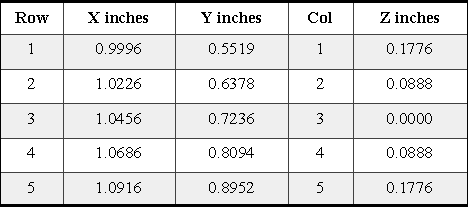ULYSSES

Ulysses HISCALE Data Analysis Handbook

## Appendix 9  Geometric Factor Study for the Deflected and Unscattered Electrons of HISCALE (Buckley MS Thesis)

Below is a figure showing how the detector is broken down into smaller area elements. The starting coordinates for the trajectories for a particular area element are located at the center of each square. The coordinates are in units of inches. The z-coordinate is the same as that shown in Figure A9-19, but y' is in the plane of the detector, and it makes approximately a 25° angle with respect to the actual y-axis.

The area of each square is .00789 in2, and the area of each partial square is .00592 in2.

Figure A9-20 Geometry of detector segmentationTable A9-7  Coordinates of detector segmentation